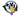# Two Simple Math Puzzles: Prime Numbers and Shortest Path

Here are two math puzzles, solve, comment and enjoy the discussion!

## Puzzle 1: Prime Numbers

Prove that $p^2-1$ is divisible by 24, where $p$ is a prime number with $p>3$.

This is a simple one - do not go by the technicality of the problem statement.

### Solution

$p^2-1 = (p-1)\times (p+1)$ Given, $p$ is a prime number $>3$, $p-1$ and $p+1$ are even. We can also write,

$p-1=2K, \text{and } p+1=2K+2=2(K+1)$

Given, $K \in \mathbb{N}$, either $K$ or $K+1$ are also even. Hence, $(p-1)\times (p+1)$ is divisible by $2\times 4 = 8$.

Furthermore, as $p$ is prime, we can write it as either $p = 3s+1$ or $p = 3s-1$, where $s \in \mathbb{N}$. In either case, one of $p-1$ or $p+1$ are divisible by 3 as well.

Hence, $p^2-1$ is divisible by $8\times 3 = 24$.

## Puzzle 2: Hopping on a Chess Board

On a chess board, basically a $8\times 8$ grid, how many ways one can go from the left most bottom corner to the right most upper corner? In chess naming conventions, from $a1$ to $h8$.

In the original post this problem was ill defined.

Please solve this problem with the constraints that only up and right moves are allowed.

Can you find the generic answer for the case of $N\times M$ grid?

### Solution

Correction:

For an $N\times M$ grid, we need only $N-1$ right and $M-1$ up moves.

Thank you Devin for pointing this out.

Given only forward moves are allowed, for any arbitrary grid of $N\times M$, a total of $(N-1) + (M-1)$ moves are needed.

Any $N-1$ of these moves can be of type right and $M-1$ of type up. In short, this is a combinatorial problem of distributing $N+M-2$ objects into groups of $N-1$ and $M-1$. This is simply,

$\dbinom{N+M-2}{N-1} = \frac{(N+M-2)!}{(N-1)! (M-1)!}$

In the particular case of the chess board, $N = M = 8$. Hence, total number of possible paths are:

$\text{No. of Paths} = \frac{14!}{7! 7!} = 3432$

Thank you Rohit and Amber for posting quick solutions!

Let me know if you have any other interesting alternative solutions to these problems.

Share This:

## Comments

### Stay in touch

Like the posts you see here? Sign up to get notified about new ones.

# Get The Best Of Posts Delivered To Your Inbox

Subscribe to my newsletter and stay updated.